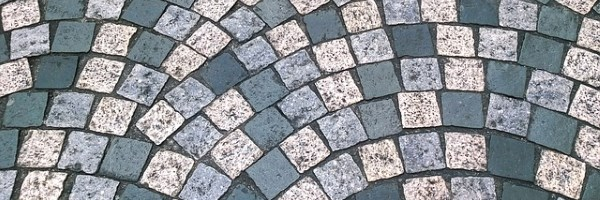For this task, you will either need multilink cubes or these sets of number base sheets to cut out: Base Three Base Four Base Five Base Six.

In the video below, Charlie and Becky show how you can make rectangles using sets of squares, sticks and units.

Can you make a rectangle to represent $x^2 + 7x + 12$?
Can you do it in more than one base?

Watch the video below to see how Charlie and Becky tackled this question:

Take 1 square, 12 sticks, and some units in your chosen base, and make some rectangles that will work in all bases.

Charlie and Becky made $x^2 + 7x + 12$ into a rectangle with length $x+4$ and width $x+3$.

Imagine you had 1 square, 100 sticks and lots of units. What can you say about the dimensions of the rectangles it is possible to make?

Now take 1 square, some sticks, and 12 units in your chosen base, and make some rectangles that will work in all bases.

Imagine you had 1 square, lots of sticks and 100 units. What can you say about the dimensions of the rectangles it is possible to make?

If you had 1 square, $p$ sticks and $q$ units, what can you say about the dimensions of the rectangles it is possible to make?

Extension

Think about the rectangles it's possible to make if you use two, three, four... squares, some sticks and some units.

Many thanks to Paul Andrews whose ideas inspired this problem.